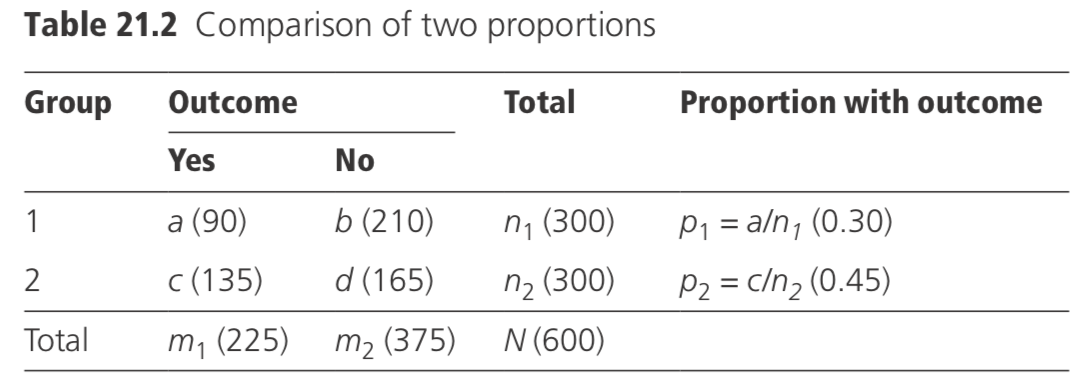# 21.4: Analysis of proportions

## 4.1 Confidence interval for a single proportion

Methods appropriate for the analysis of proportions are used when the outcome of interest is a binary (‘yes/no’) variable (for example, the proportion of individuals who develop a disease). The standard error of a proportion p, calculated from a sample of nsubjects, is estimated as $$√[ p(1− p)/n]$$. For example, if the prevalence of splenomegaly in a random sample of 200 children from a population is found to be 0.40 (40%) (i.e. 80 had splenomegaly), the standard error (SE) is given by:

$\mathrm{SE}(p)=\sqrt{(0.40 \times 0.60 / 200)}=0.035(3.5 \%)$

The 95% CI for a proportion is given by $$p±1.96×\text{SE}(p)$$.In the example, the 95% CI is 0.4±1.96×0.035 or (0.33, 0.47), i.e. 33–47%. There is a 95% chance that the true prevalence of splenomegaly in the population from which the sample of 200 was taken was between 33% and 47%.

## 4.2 Difference between two proportions

Suppose now that the objective is to compare the proportions observed in two groups of individuals, as is typically the case in a trial, comparing outcomes in an intervention and control group. The standard error of the difference between two proportions $$p_1$$ and $$p_2$$, based on $$n_1$$ and $$n_2$$ observations, respectively, is estimated approximately as:

$\sqrt{\langle }\left\{\overline{\mathrm{p}}(1-\overline{\mathrm{p}})\left[\left(1 / n_{1}\right)+\left(1 / n_{2}\right)\right]\right\}$

where $$\bar{p}=(n_1p_1+n_2p_2)/(n_1+n_2).$$
For example, if the proportions to be compared are 90/300 (30%) and 135/300

(45%), the observed difference between the two proportions is −0.15, p̄ = 0.375, and the standard error of the difference is given by:

$\sqrt{\{ }(0.375 \times 0.625[(1 / 300)+(1 / 300)]\}=0.040$

The 95% CI for the difference between the proportions is given by(p1 – p2 )±1.96×SE.In the example, this gives (−0.15)±1.96(0.040), i.e. (−0.23, −0.07), or −23% to −7%.

To test the null hypothesis that there is no true difference between the two proportions, the data are first arranged in a 2 × 2 table, as in Table 21.2.

In the table, a is the number in group 1 who experiences the outcome of interest. The expected value of a, E(a), and the variance of a, V(a), are calculated under the hypothesis of no difference between the two groups:

$E(a)=m_{1} n_{1} / N$$V(a)=n_{1} n_{2} m_{1} m_{2} /\left[N^{2}(N-1)\right]$

The chi-squared (χ2 ) statistic is then calculated. This gives a measure of the extent to which the observed data differ from those expected if the two proportions were truly equal.

$\chi^{2}=(|a-E(a)|-0.5)^{2} / V(a)$

where |aE(a)| indicates the absolute value of [aE(a)].

The calculated value of χ 2 is compared with tables of the chi-squared distribution

with one degree of freedom (df). If it exceeds 3.84, then p < 0.05, indicating some evidence of a real difference in the proportions. If it exceeds 6.63, then p < 0.01, and there is strong evidence of a difference.

In the example, a = 90; E(a) = (225)(300)/600 = 112.50; and V(a) = (300 × 300 × 22522 × 375)/(600 × 600 × 599) = 35.215. Thus $$\chi^2=(|90−112.50|−0.5)2/35.212=13.74$$. From tables of the chi-squared distribution, a p-value of 0.0002 is obtained, indicating a difference as large as that observed would be very unlikely to arise by chance if there really was no difference between the two groups.

If any of the quantities E(a), E(b), E(c), or E(d) (for example,$$E(b)=m_2n_2/N$$ ) are less than 5.0 and N is less than 40, the χ2 test is invalid, and a test called ‘Fisher’s exact test’ should be used instead (Kirkwood and Sterne, 2003).

## 4.3 Ratio of two proportions

The ratio of two proportions is sometimes referred to as the relative risk (R). To construct a CI for a relative risk, the natural logarithm of the estimate of the relative risk is computed (Table 21.2):

$\log _{e}(R)=\log _{e}\left(p_{1} / p_{2}\right)=\log _{e}\left[\left(a / n_{1}\right) /\left(c / n_{2}\right)\right]$

Its standard error is estimated by:

$\operatorname{SE}\left[\log _{e}(R)\right]=\sqrt{\{ }\left[b /\left(a n_{1}\right)\right]+\left[d /\left(c n_{2}\right)\right] \}$

The 95% CI for loge(R) is given by $$\log_e (R)±1.96$$ SE, and the 95% CI for the relative risk is obtained by taking anti-logarithms.

In the example given in Table 21.2, the relative risk is estimated as $$0.30/0.45 = 0.667$$, and $$\log _e(R)=−0.405$$. The SE[loge(R)] is estimated as √{[210/(90 × 300)] + [165/ (135 × 300)]} = 0.109, and the 95% CI for loge(R) is given by −0.405 ± 1.96(0.109), i.e. (−0.619, −0.191). Taking anti-logarithms, the 95% CI for the relative risk is (0.538, 0.826).

## 4.4 Trend test for proportions

Sometimes, it is of interest to examine whether there is a trend in a series of proportions associated with different levels of some underlying characteristic. For example, consider the proportion of leprosy patients who report regularly to collect their monthly drug supply from a clinic when the accessibility of the clinic is rated as very poor, poor, fair, or good (Table 21.3).

A ‘score’ (xi) is assigned for each kind of clinic, of which the value relates to the level of accessibility. For example, ‘0’ has been assigned to those with ‘very poor’ accessibility and ‘3’ to those with ‘good’ accessibility. A test for the trend in the proportions $$a_1/n_1, a_2/n_2, a_3/n_3,$$ and $$a_4/n_4$$ is provided by testing, as a chi-squared with one df, the expression:

$\chi^{2}=N\left[\left(N \Sigma a_{1} x_{\mathrm{i}}\right)-\left(A \Sigma n_{\mathrm{i}} x_{\mathrm{i}}\right)\right]^{2} /\left\{A(N-A)\left[\left(N \Sigma n_{\mathrm{i}} x_{\mathrm{i}}^{2}\right)-\left(\Sigma n_{\mathrm{i}} x_{\mathrm{i}}\right)^{2}\right]\right\}$

For example, suppose the data are as shown in Table 21.3 (the respective percentages of regular attenders in the four rows are 20%, 30%, 50%, and 60%). The value of χ2 is:

$150[(150×125)−(63×245)]^2/{63×87[(150×555)−245^2]}=12.95$

which is highly significant (p = 0.0003), based on a χ2 test with one df. It may be concluded therefore that there is strong evidence that the regularity of drug collection increases with the accessibility of the clinic.